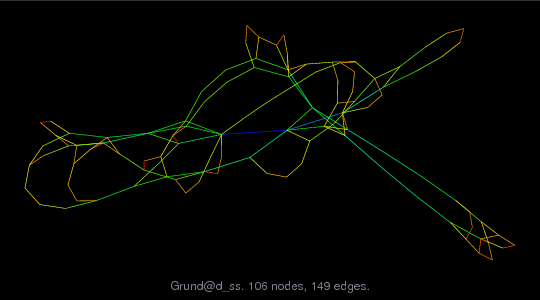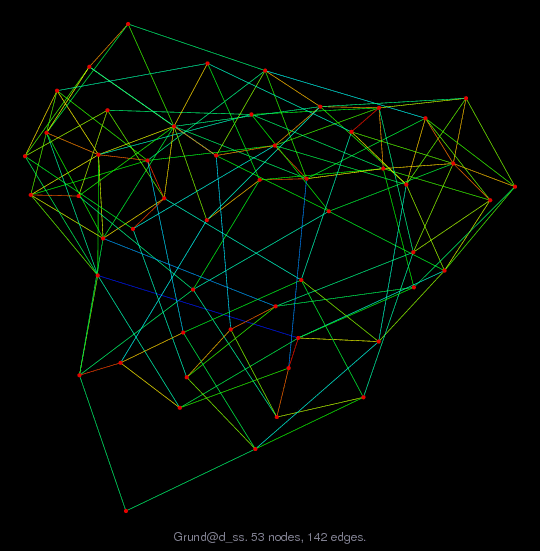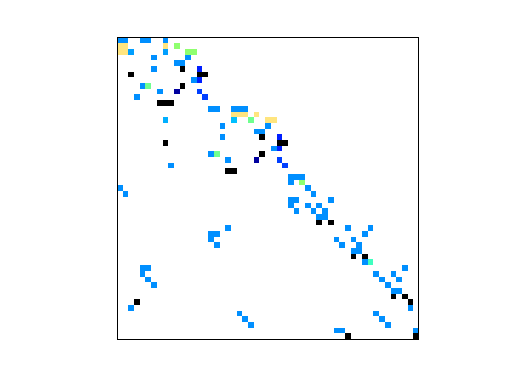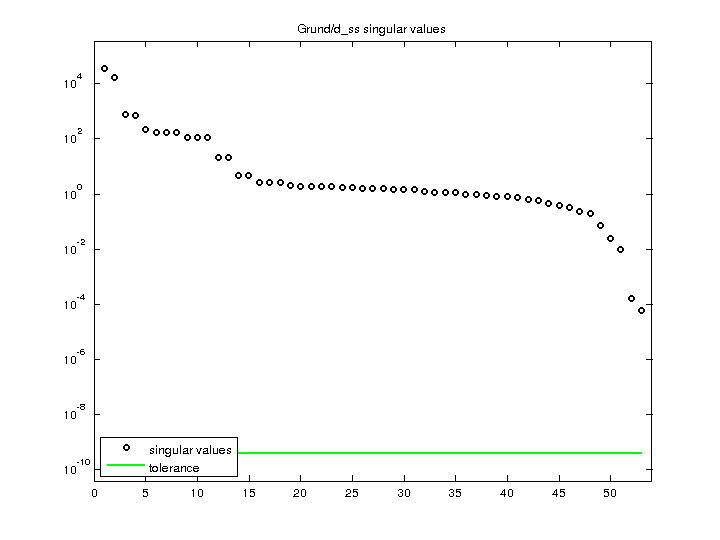Matrix: Grund/d_ss

Description: Unsymmetric Matrix d_ss, F. Grund, Dec 1994.(bipartite graph drawing) (graph drawing of A+A')• Matrix group: Grund
• download as a MATLAB mat-file, file size: 1 KB. Use UFget(464) or UFget('Grund/d_ss') in MATLAB.

 Matrix properties number of rows 53 number of columns 53 nonzeros 144 structural full rank? yes structural rank 53 # of blocks from dmperm 1 # strongly connected comp. 1 explicit zero entries 5 nonzero pattern symmetry 4% numeric value symmetry 0% type real structure unsymmetric Cholesky candidate? no positive definite? no

 author F. Grund editor F. Grund date 1997 kind chemical process simulation problem 2D/3D problem? no

 Additional fields size and type b full 53-by-1

 Ordering statistics: result nnz(chol(P*(A+A'+s*I)*P')) with AMD 474 Cholesky flop count 5.5e+03 nnz(L+U), no partial pivoting, with AMD 895 nnz(V) for QR, upper bound nnz(L) for LU, with COLAMD 139 nnz(R) for QR, upper bound nnz(U) for LU, with COLAMD 255

Note that all matrix statistics (except nonzero pattern symmetry) exclude the 5 explicit zero entries.

 SVD-based statistics: norm(A) 35698.9 min(svd(A)) 5.80955e-05 cond(A) 6.14486e+08 rank(A) 53 sprank(A)-rank(A) 0 null space dimension 0 full numerical rank? yes

 singular values (MAT file): click here SVD method used: s = svd (full (A)) ; status: ok